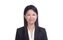cancel
Showing results for
Did you mean:Frequent Visitor

## Optimize measure

Hi,

I have a lot of measures on my report to calculate totals by the max date. Here is one of my measure-

``````mUnitsTotal =
VAR MaxTable =
SUMMARIZE('Sales','Sales'[Job],'Sales'[SubJob],"vDate",MAX('Sales'[EntryDates])),
"BudgetedUnits",
MAXX(
FILTER(ALL('Sales'),[Job]=EARLIER([Job]) && 'Sales'[SubJob]=EARLIER('Sales'[SubJob]) && [EntryDates]=[vDate]),
[Unit Total])
)
RETURN
SUMX(MaxTable,[BudgetedUnits])``````

Is this an optimized measure? If not, please suggest alternatives.

At the moment the dataset has a few thousand rows with entries for each day by Job and SubJob. Eventually the dataset would grow exponentially as we add more jobs.

The reports will be filtered by job by the end users and measures should respond fairly quickly. Please advise.

1 ACCEPTED SOLUTIONCommunity Support

Hi @SR11

The performance of EARLIER might be slow, depending on the syntax of the expression, it may perform multiple operations. For example if you have 10 rows in the column, approximately a 100 operations could be required. Therefore, It should be avoided as much as possible. You can try this Measure as its replace.

``````mUnitsTotal =

VAR max_date =

Sales,

"max_date",

CALCULATE (

MAX ( Sales[EntryDates] ),

ALLEXCEPT ( Sales, Sales[Job], Sales[SubJob] )

)

)

VAR mTotal =

SUMX ( FILTER ( max_date, [max_date] = [EntryDates] ), [Unit Total] )

RETURN

IF ( HASONEFILTER ( Sales[EntryDates] ), MAX ( Sales[Unit Total] ), mTotal )``````

The result looks like this:For more details, you can refer the attached pbix file.

Best Regards

Caiyun Zheng

If this post helps, please consider make it as the solution by Accept it as Solution. Really appreciate!

3 REPLIES 3Frequent Visitor

Thanks for the feedback. I will work on updating the measure. Thanks again!Super User

Hi @SR11

SUMMARIZE has performance issue, you can read some articles like this one: All the secrets of SUMMARIZE - SQLBI

Normally you can use GROUPBY and ADDCOLUMNS to achieve the same thing, sometimes GENERATE ROW as well.

I am not sure if you might have more than 1 entry for the same vDate, but I guess yes, according to your measure, so I modified the one from @v-cazheng-msft a little bit as below. You can check the performance in DAX Studio.

``````mUnitsTotal =
VAR T1 =
Sales,
"vdate",
CALCULATE (
MAX ( Sales[EntryDates] ),
ALLEXCEPT ( Sales, Sales[Job], Sales[SubJob] )
)
)
VAR T2 =
GROUPBY (
FILTER ( T1, [EntryDates] = [vdate] ),
Sales[Job],
Sales[SubJob],
"BudgetedUnits", MAXX ( CURRENTGROUP (), [Unit Total] )
)
RETURN
SUMX ( T2, [BudgetedUnits] )``````Community Support

Hi @SR11

The performance of EARLIER might be slow, depending on the syntax of the expression, it may perform multiple operations. For example if you have 10 rows in the column, approximately a 100 operations could be required. Therefore, It should be avoided as much as possible. You can try this Measure as its replace.

``````mUnitsTotal =

VAR max_date =

Sales,

"max_date",

CALCULATE (

MAX ( Sales[EntryDates] ),

ALLEXCEPT ( Sales, Sales[Job], Sales[SubJob] )

)

)

VAR mTotal =

SUMX ( FILTER ( max_date, [max_date] = [EntryDates] ), [Unit Total] )

RETURN

IF ( HASONEFILTER ( Sales[EntryDates] ), MAX ( Sales[Unit Total] ), mTotal )``````

The result looks like this:For more details, you can refer the attached pbix file.

Best Regards

Caiyun Zheng

If this post helps, please consider make it as the solution by Accept it as Solution. Really appreciate!